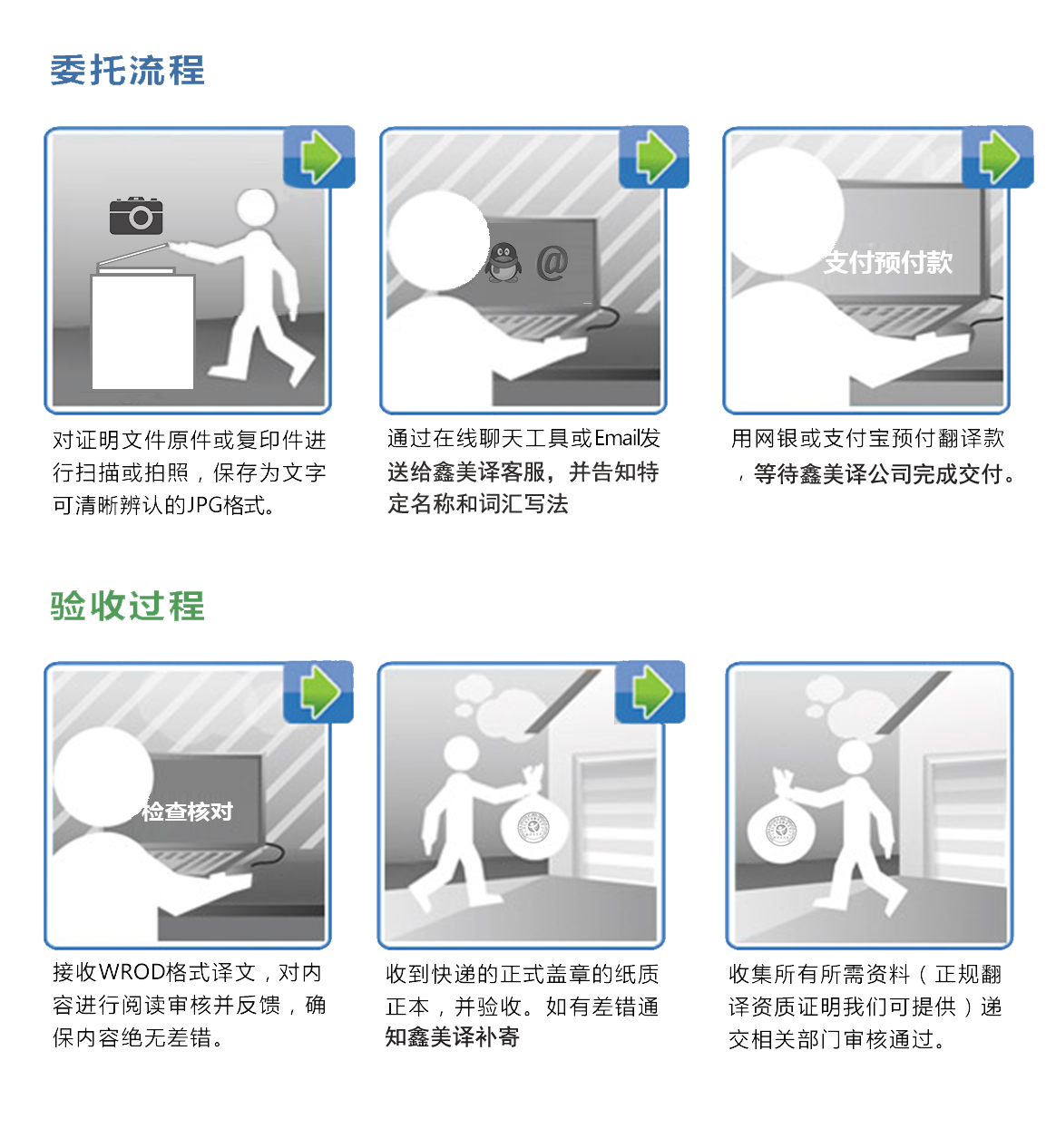﻿ 厦门证件翻译公司_有资质证书翻译机构-厦门鑫美译公司## 证明翻译机构资质

１．专业国家工商局注册翻译机构。
２．中文公司全称须有“翻译”字样，不能以“咨询服务、文化传播、科技发展、信息技术公司”等盖章。
３．英文公司全称须有“Translation”字样。
４．拥有备案编码。

## 证件翻译盖章价格参考

 证件翻译报价 证件类型 语种 备注 本列报价仅针对英文资料 其他语种请来电咨询 英语 日语、韩语 德语、法语、俄语 西、葡、意、阿 蒙、泰、越、缅、柬等小语种 毕业证 100 120+ 150+ 180+ 200+ 学位证 80-120 100-150+ 100-200+ 150+ 200+ 一张学位证+一张成绩单=200元，成绩单多一页增加50-100元 （中文成绩单200元） 成绩单 100-150 100-200+ 200+ 200+ 200+ 学历证明 100+ 150+ 150+ 200+ 200+ 在读证明 100+ 130+ 150+ 200+ 200+ 录取通知书 100+ 180+ 200+ 200+ 200+ 护照 100+ 130+ 150+ 200+ 200+ 出生证明 100+ 180+ 200+ 200+ 200+ 无犯罪记录证明 100+ 130+ 150+ 200+ 200+ 婚姻证明 100+ 130+ 150+ 200+ 200+ 职业证明 100+ 130+ 150+ 200+ 200+ 户口本 50+ 130+ 150+ 200+ 200+ 签证 100+ 150+ 150+ 200+ 200+ 驾驶证 100+ 150+ 150+ 200+ 200+ 结婚证 100+ 130+ 150+ 200+ 200+ 身份证 100+ 130+ 150+ 200+ 200+ 营业执照 150+ 180+ 200+ 200+ 200+ 税务本 100+ 130+ 150+ 200+ 200+

## 证件翻译盖章常见问题### 证件证书翻译

• 文章目录

18950110895
QQ在线咨询

#### 微信•本页二维码18950110895